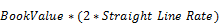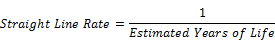# Double Declining Balance Depreciation - HxGN EAM - Version 11.07.01 - Feature Briefs - Hexagon

## HxGN EAM Equipment Depreciation

Language
English
Product
HxGN EAM
Search by Category
Feature Briefs
HxGN EAM Version
11.7.1

The double declining balance depreciation method is like an accelerated version of straight-line depreciation. To use it, first calculate depreciation like using the straight line method. You then figure out the total percentage of the asset that is depreciated the first year and double it. Each subsequent year, that same percentage is multiplied by the remaining balance to be depreciated. At some point, the value will be lower than the straight-line charge, at which point, the double declining method will be scrapped, and straight line used for the remainder of the asset’s life.Example: In the straight-line example, we calculated that a \$5000 machine with a \$200 salvage value and an estimated useful life of five years would be depreciated by \$960 annually. The first year, we must compare this to the total amount to be depreciated, in this case, \$4800 (\$5000 base - \$200 salvage value = \$4800). Dividing \$960 by \$4800, we determine the straight-line depreciation charge is 20% of the total depreciation amount of \$4800. Using this information, the figure is doubled to 40%. So, in the first year, you take \$4800 multiplied by 40% to get a total depreciation charge of \$1920. In the second year, you take the same percentage (40%) and multiply it by the remaining amount to be depreciated (\$4800 - \$1920 = \$2880) to get \$1152. In the third year, the remaining amount to be depreciated is \$1728 (\$2880 - \$1152 = \$1728), multiplied by 40% yields a depreciation charge of \$691.20. However, this figure (\$691.20) is below the amount that would be charged using straight line depreciation and so the double declining balance is, and straight line immediately used. Therefore, the depreciation charge in the third year is \$960.